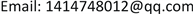﻿ 一类传染病模型在有向网络上的传播阈值与免疫策略研究 Study on the Spread Threshold and Immunization Scheme of a Class of Infectious Disease Model on Directed Networks

Pure Mathematics
Vol. 09  No. 02 ( 2019 ), Article ID: 29335 , 8 pages
10.12677/PM.2019.92026

Study on the Spread Threshold and Immunization Scheme of a Class of Infectious Disease Model on Directed Networks

Wei Shi

School of Science, Shanghai University, ShanghaiReceived: Feb. 27th, 2019; accepted: Mar. 13th, 2019; published: Mar. 20th, 2019ABSTRACT

This paper extends the traditional SIS model with birth and death to a directed scale-free network, calculates the endemic equilibrium point and critical epidemic threshold of the model under constant and linear node’s infectivity, and puts three traditional immunization schemes on the model, which rigorously proved that under the three immunization strategies, the model’s inhibition of infectious diseases increased. Further comparing the performance of three different immunization strategies under the same average immunization rate, the target immunization of immunizing nodes with large in-degrees and out-degrees is more effective than proportional and acquaintance immunization.

Keywords:Scale-Free Network, SIS Model, Epidemic Threshold, Immunization Strategy1. 引言

$\left\{\begin{array}{l}\frac{\text{d}{x}_{k}\left(t\right)}{\text{d}t}=\delta \left(1-{x}_{k}-{y}_{k}\right)-\lambda k{x}_{k}{\Theta }_{k}\left({y}_{k}\right)-\alpha {x}_{k}+\gamma {y}_{k},\hfill \\ \frac{\text{d}{y}_{k}\left(t\right)}{\text{d}t}=\lambda k{x}_{k}{\Theta }_{k}\left({y}_{k}\right)-\left(\beta +\gamma \right){y}_{k}.\hfill \end{array}$

2. 传染病模型及流行病阈值

$\left\{\begin{array}{l}\frac{\text{d}{S}_{k,l}\left(t\right)}{\text{d}t}=b\left(1-{S}_{k,l}\left(t\right)-{I}_{k,l}\left(t\right)\right)-\lambda k{S}_{k,l}\left(t\right)\Theta \left(t\right)-\mu {S}_{k,l}\left(t\right)+\gamma {I}_{k,l}\left(t\right),\hfill \\ \frac{\text{d}{I}_{k,l}\left(t\right)}{\text{d}t}=\lambda k{S}_{k,l}\left(t\right)\Theta \left(t\right)-\left(\beta +\gamma \right){I}_{k,l}\left(t\right),\hfill \end{array}$ (2.1)

$\Theta \left(t\right)=\frac{{\sum }_{k,l}\varphi \left(k,l\right)p\left(k,l\right){I}_{k,l}\left(t\right)}{{\sum }_{k,l}lp\left(k,l\right)}=\frac{{\sum }_{k,l}\varphi \left(k,l\right)p\left(k,l\right){I}_{k,l}\left(t\right)}{〈l〉},$ (2.2)

$〈k〉=\underset{k,l}{\sum }kp\left(k,l\right)=\underset{k}{\sum }kP\left(k\right)\text{ },\text{\hspace{0.17em}}〈l〉=\underset{k,l}{\sum }lp\left(k,l\right)=\underset{l}{\sum }lQ\left(l\right)\text{ }\text{ }.$

$\left\{\begin{array}{l}b\left(1-{S}_{k,l}\left(t\right)-{I}_{k,l}\left(t\right)\right)-\lambda k{S}_{k,l}\left(t\right)\Theta \left(t\right)-\mu {S}_{k,l}\left(t\right)+\gamma {I}_{k,l}\left(t\right)=0,\hfill \\ \lambda k{S}_{k,l}\left(t\right)\Theta \left(t\right)-\left(\beta +\gamma \right){I}_{k,l}\left(t\right)=0.\hfill \end{array}$ (2.3)

$\left\{\begin{array}{l}{S}_{k,l}^{*}=\frac{b\left(\beta +\gamma \right)}{\left(\beta +\gamma \right)\left(b+\mu \right)+\left(b+\beta \right)\lambda k\Theta },\hfill \\ {I}_{k,l}^{*}=\frac{b\lambda k\Theta }{\left(\beta +\gamma \right)\left(b+\mu \right)+\left(b+\beta \right)\lambda k\Theta }.\hfill \end{array}$ (2.4)

$\Theta \left(t\right)=\frac{1}{〈l〉}\underset{k,l}{\sum }\varphi \left(k,l\right)p\left(k,l\right)\frac{b\lambda k\Theta }{\left(\beta +\gamma \right)\left(b+\mu \right)+\left(b+\beta \right)\lambda k\Theta }.$ (2.5)

$\Theta \left(t\right)=\frac{1}{〈l〉}\underset{k,l}{\sum }\varphi \left(k,l\right)p\left(k,l\right)\frac{b\lambda k\Theta }{\left(\beta +\gamma \right)\left(b+\mu \right)+\left(b+\beta \right)\lambda k\Theta }\triangleq f\left(\Theta \right).$ (2.6)

$\begin{array}{l}f\left(1\right)=\frac{1}{〈l〉}\underset{k,l}{\sum }\varphi \left(k,l\right)p\left(k,l\right)\frac{b\lambda k}{\left(\beta +\gamma \right)\left(b+\mu \right)+\left(b+\beta \right)\lambda k\Theta }<\frac{1}{〈l〉}\underset{k,l}{\sum }lp\left(k,l\right)=1,\\ \text{}{f}^{\prime }\left(\Theta \right)=\frac{1}{〈l〉}\underset{k,l}{\sum }\varphi \left(k,l\right)p\left(k,l\right)\frac{b\lambda k\left(\beta +\gamma \right)\left(b+\mu \right)}{{\left[\left(\beta +\gamma \right)\left(b+\mu \right)+\left(b+\beta \right)\lambda k\Theta \right]}^{2}}>0,\\ {f}^{″}\left(\Theta \right)=\frac{1}{〈l〉}\underset{k,l}{\sum }\varphi \left(k,l\right)p\left(k,l\right)\frac{-2b\left(\beta +\gamma \right)\left(b+\mu \right)\left(b+\beta \right){\lambda }^{2}{k}^{2}}{{\left[\left(\beta +\gamma \right)\left(b+\mu \right)+\left(b+\beta \right)\lambda k\Theta \right]}^{3}}<0;\end{array}$

$\frac{\text{d}f\left(\Theta \right)}{\text{d}\Theta }={\frac{\text{d}}{\text{d}\Theta }\left(\frac{1}{〈l〉}\underset{k,l}{\sum }\varphi \left(k,l\right)p\left(k,l\right)\frac{b\lambda k\Theta }{\left(\beta +\gamma \right)\left(b+\mu \right)+\left(b+\beta \right)\lambda k\Theta }\right)|}_{\Theta =0}>1.$ (2.7)

 (2.8)

3. 免疫策略

$\left\{\begin{array}{l}\frac{\text{d}{S}_{k,l}\left(t\right)}{\text{d}t}=b\left(1-{S}_{k,l}\left(t\right)-{I}_{k,l}\left(t\right)\right)-\lambda k\left(1-{\delta }_{k,l}\right){S}_{k,l}\left(t\right)\Theta \left(t\right)-\mu {S}_{k,l}\left(t\right)+\gamma {I}_{k,l}\left(t\right),\hfill \\ \frac{\text{d}{I}_{k,l}\left(t\right)}{\text{d}t}=\lambda k\left(1-{\delta }_{k,l}\right){S}_{k,l}\left(t\right)\Theta \left(t\right)-\left(\beta +\gamma \right){I}_{k,l}\left(t\right).\hfill \end{array}$ (3.1)

$\left\{\begin{array}{l}{S}_{k,l}^{*}=\frac{b\left(\beta +\gamma \right)}{\left(\beta +\gamma \right)\left(b+\mu \right)+\left(b+\beta \right)\lambda k\left(1-{\delta }_{k,l}\right)\Theta },\hfill \\ {I}_{k,l}^{*}=\frac{b\lambda k\left(1-{\delta }_{k,l}\right)\Theta }{\left(\beta +\gamma \right)\left(b+\mu \right)+\left(b+\beta \right)\lambda k\left(1-{\delta }_{k,l}\right)\Theta }.\hfill \end{array}$ (3.2)

$\frac{\text{d}\stackrel{¯}{f}\left(\Theta \right)}{\text{d}\Theta }={\frac{\text{d}}{\text{d}\Theta }\left(\frac{1}{〈l〉}\underset{k,l}{\sum }\varphi \left(k,l\right)p\left(k,l\right)\frac{b\lambda k\left(1-{\delta }_{k,l}\right)\Theta }{\left(\beta +\gamma \right)\left(b+\mu \right)+\left(b+\beta \right)\lambda k\left(1-{\delta }_{k,l}\right)\Theta }\right)|}_{\Theta =0}>1.$ (3.3)(3.4)

3.1. 比例免疫

${\stackrel{¯}{\lambda }}_{c}=\frac{〈l〉\left(\beta +\gamma \right)\left(b+\mu \right)}{b\left(〈k\varphi \left(k,l\right)〉-〈k\varphi \left(k,l\right)\delta 〉\right)}=\left\{\begin{array}{l}\frac{\left(\beta +\gamma \right)\left(b+\mu \right)}{ab〈k〉\left(1-\delta \right)},\text{}\varphi \left(k,l\right)=al,\hfill \\ \frac{\left(\beta +\gamma \right)\left(b+\mu \right)}{bA\left(1-\delta \right)},\text{}\varphi \left(k,l\right)=A,\hfill \end{array}=\frac{1}{1-\delta }{\lambda }_{c}.$ (3.5)

3.2. 熟人免疫

$\begin{array}{c}{\stackrel{^}{\lambda }}_{c}=\frac{〈l〉\left(\beta +\gamma \right)\left(b+\mu \right)}{b\left(〈k\varphi \left(k,l\right)〉-p〈{k}^{2}\varphi \left(k,l\right)P\left(k\right)〉/〈k〉\right)}\\ =\left\{\begin{array}{l}\frac{\left(\beta +\gamma \right)\left(b+\mu \right)}{ab\left(〈k〉-p〈{k}^{2}P\left(k\right)〉/〈k〉\right)},\text{}\varphi \left(k,l\right)=al,\hfill \\ \frac{〈l〉\left(\beta +\gamma \right)\left(b+\mu \right)}{bA\left(〈k〉-p〈{k}^{2}P\left(k\right)〉/〈k〉\right)},\text{}\varphi \left(k,l\right)=A,\hfill \end{array}\\ =\left\{\begin{array}{l}\frac{1}{1-p〈{k}^{2}P\left(k\right)〉/{〈k〉}^{2}}{\lambda }_{c},\text{}\varphi \left(k,l\right)=al,\hfill \\ \frac{〈l〉}{〈k〉-p〈{k}^{2}P\left(k\right)〉/〈k〉}{\lambda }_{c},\text{}\varphi \left(k,l\right)=A.\hfill \end{array}\end{array}$ (3.6)

$\begin{array}{l}\frac{1}{1-p〈{k}^{2}P\left(k\right)〉/{〈k〉}^{2}}>\frac{1}{1-p〈{k}^{2}P\left(k\right)〉/〈{k}^{2}〉}>1,\\ \frac{〈l〉}{〈k〉-p〈{k}^{2}P\left(k\right)〉/〈k〉}=\frac{1}{1-p〈{k}^{2}P\left(k\right)〉/{〈k〉}^{2}}>1.\end{array}$

3.3. 目标免疫(3.7)

${\stackrel{˜}{\delta }}_{k,l}$ 代入方程(3.4)，我们可以得到目标免疫的传播阈值：

${\stackrel{˜}{\lambda }}_{c}=\frac{〈l〉\left(\beta +\gamma \right)\left(b+\mu \right)}{b\left(〈k\varphi \left(k,l\right)〉-〈k\varphi \left(k,l\right){\stackrel{˜}{\delta }}_{k,l}〉\right)}=\left\{\begin{array}{l}\frac{〈l〉\left(\beta +\gamma \right)\left(b+\mu \right)}{ab\left(〈kl〉-〈kl{\stackrel{˜}{\delta }}_{k,l}〉\right)}\hfill \\ \frac{〈l〉\left(\beta +\gamma \right)\left(b+\mu \right)}{bA\left(〈k〉-〈k{\stackrel{˜}{\delta }}_{k,l}〉\right)}\hfill \end{array}=\left\{\begin{array}{l}\frac{{〈l〉}^{2}}{{〈l〉}^{2}-〈kl{\stackrel{˜}{\delta }}_{k,l}〉}{\lambda }_{c},\text{}\varphi \left(k,l\right)=al,\hfill \\ \frac{〈l〉}{〈k〉-〈k{\stackrel{˜}{\delta }}_{k,l}〉}{\lambda }_{c},\text{}\varphi \left(k,l\right)=A.\hfill \end{array}$ (3.8)

$〈kl{\stackrel{˜}{\delta }}_{k,l}〉=\alpha 〈kl〉$ ，其中 $0<\alpha <1$$〈k{\stackrel{˜}{\delta }}_{k,l}〉=\beta 〈k〉$ ，其中 $0<\beta <1$ 。所以

$\begin{array}{l}\frac{{〈l〉}^{2}}{{〈l〉}^{2}-〈kl{\stackrel{˜}{\delta }}_{k,l}〉}=\frac{{〈l〉}^{2}}{{〈l〉}^{2}\left(1-\alpha \right)}>1,\\ \frac{〈l〉}{〈k〉-〈k{\stackrel{˜}{\delta }}_{k,l}〉}=\frac{〈l〉}{〈l〉\left(1-\beta \right)}>1.\end{array}$

4. 免疫策略对比

4.1. 目标免疫与比例免疫

$\begin{array}{l}{\stackrel{˜}{\lambda }}_{c}-{\stackrel{¯}{\lambda }}_{c}\\ =\frac{〈l〉\left(\beta +\gamma \right)\left(b+\mu \right)}{b\left(〈k\varphi \left(k,l\right)〉-〈k\varphi \left(k,l\right){\stackrel{˜}{\delta }}_{k,l}〉\right)}-\frac{〈l〉\left(\beta +\gamma \right)\left(b+\mu \right)}{b\left(〈k\varphi \left(k,l\right)〉-〈k\varphi \left(k,l\right)\delta 〉\right)}\\ =\frac{〈l〉\left(\beta +\gamma \right)\left(b+\mu \right)}{b}\left(\frac{1}{〈k\varphi \left(k,l\right)〉-〈\varphi \left(k,l\right)〉〈k{\stackrel{˜}{\delta }}_{k,l}〉}-\frac{1}{〈k\varphi \left(k,l\right)〉-〈\varphi \left(k,l\right)〉〈k\delta 〉}\right)\end{array}$ (4.1)

$\begin{array}{c}〈k{\stackrel{˜}{\delta }}_{k,l}〉-〈k\delta 〉=\underset{k=\kappa }{\overset{M}{\sum }}kP\left(k\right)\underset{l=\tau }{\overset{N}{\sum }}Q\left(l\right)-\underset{k=\kappa }{\overset{M}{\sum }}P\left(k\right)\underset{l=\tau }{\overset{N}{\sum }}Q\left(l\right)\underset{k=1}{\overset{M}{\sum }}kP\left(k\right)\\ =\underset{l=\tau }{\overset{N}{\sum }}Q\left(l\right)\left(\underset{k=\kappa }{\overset{M}{\sum }}kP\left(k\right)-\underset{k=\kappa }{\overset{M}{\sum }}P\left(k\right)\underset{k=1}{\overset{M}{\sum }}kP\left(k\right)\right)\\ =\underset{l=\tau }{\overset{N}{\sum }}Q\left(l\right)\left(\underset{k=\kappa }{\overset{M}{\sum }}kP\left(k\right)-\underset{k=\kappa }{\overset{M}{\sum }}P\left(k\right)\left(\underset{k=1}{\overset{\kappa -1}{\sum }}kP\left(k\right)+\underset{k=\kappa }{\overset{M}{\sum }}kP\left(k\right)\right)\right)\\ =\underset{l=\tau }{\overset{N}{\sum }}Q\left(l\right)\left(\underset{k=\kappa }{\overset{M}{\sum }}kP\left(k\right)\underset{k=1}{\overset{\kappa -1}{\sum }}P\left(k\right)-\underset{k=\kappa }{\overset{M}{\sum }}P\left(k\right)\underset{k=1}{\overset{\kappa -1}{\sum }}kP\left(k\right)\right)\\ =\underset{l=\tau }{\overset{N}{\sum }}Q\left(l\right)\left(\underset{\stackrel{¯}{k}=\kappa }{\overset{M}{\sum }}\stackrel{¯}{k}P\left(\stackrel{¯}{k}\right)\underset{k=1}{\overset{\kappa -1}{\sum }}P\left(k\right)-\underset{\stackrel{¯}{k}=\kappa }{\overset{M}{\sum }}P\left(\stackrel{¯}{k}\right)\underset{k=1}{\overset{\kappa -1}{\sum }}kP\left(k\right)\right)\end{array}$

$\begin{array}{l}=\underset{l=\tau }{\overset{N}{\sum }}Q\left(l\right)\underset{\stackrel{¯}{k}=\kappa }{\overset{M}{\sum }}\underset{k=1}{\overset{\kappa -1}{\sum }}P\left(\stackrel{¯}{k}\right)P\left(k\right)\left(\stackrel{¯}{k}-k\right)\\ >0.\end{array}$ (4.2)

4.2. 目标免疫与熟人免疫

$\begin{array}{l}{\stackrel{˜}{\lambda }}_{c}-{\stackrel{^}{\lambda }}_{c}\\ =\frac{〈l〉\left(\beta +\gamma \right)\left(b+\mu \right)}{b\left(〈k\varphi \left(k,l\right)〉-〈k\varphi \left(k,l\right){\stackrel{˜}{\delta }}_{k,l}〉\right)}-\frac{〈l〉\left(\beta +\gamma \right)\left(b+\mu \right)}{b\left(〈k\varphi \left(k,l\right)〉-p〈{k}^{2}\varphi \left(k,l\right)P\left(k\right)〉/〈k〉\right)}\\ =\frac{〈l〉\left(\beta +\gamma \right)\left(b+\mu \right)}{b}\left(\frac{1}{〈k\varphi \left(k,l\right)〉-〈\varphi \left(k,l\right)〉〈k{\stackrel{˜}{\delta }}_{k,l}〉}-\frac{1}{p〈\varphi \left(k,l\right)〉〈{k}^{2}P\left(k\right)〉/〈k〉}\right)\end{array}$ (4.3)

$\begin{array}{c}〈k{\stackrel{˜}{\delta }}_{k,l}〉-p〈{k}^{2}P\left(k\right)〉/〈k〉=〈k{\stackrel{˜}{\delta }}_{k,l}〉-\frac{〈k〉〈{\stackrel{˜}{\delta }}_{k,l}〉}{〈kP\left(k\right)〉}\cdot \frac{〈{k}^{2}P\left(k\right)〉}{〈k〉}\\ =\frac{〈kP\left(k\right)〉〈k{\stackrel{˜}{\delta }}_{k,l}〉-〈{\stackrel{˜}{\delta }}_{k,l}〉〈{k}^{2}P\left(k\right)〉}{〈kP\left(k\right)〉}\end{array}$ (4.4)

$\begin{array}{l}〈kP\left(k\right)〉〈k{\stackrel{˜}{\delta }}_{k,l}〉-〈{\stackrel{˜}{\delta }}_{k,l}〉〈{k}^{2}P\left(k\right)〉\\ =\underset{k=1}{\overset{M}{\sum }}kP{\left(k\right)}^{2}\underset{k=\kappa }{\overset{M}{\sum }}kP\left(k\right)\underset{l=\tau }{\overset{N}{\sum }}Q\left(l\right)-\underset{k=\kappa }{\overset{M}{\sum }}P\left(k\right)\underset{l=\tau }{\overset{N}{\sum }}Q\left(l\right)\underset{k=1}{\overset{M}{\sum }}{k}^{2}P{\left(k\right)}^{2}\\ =\underset{l=\tau }{\overset{N}{\sum }}Q\left(l\right)\left(\underset{k=1}{\overset{M}{\sum }}kP{\left(k\right)}^{2}\underset{k=\kappa }{\overset{M}{\sum }}kP\left(k\right)-\underset{k=\kappa }{\overset{M}{\sum }}P\left(k\right)\underset{k=1}{\overset{M}{\sum }}{k}^{2}P{\left(k\right)}^{2}\right)\\ =\underset{l=\tau }{\overset{N}{\sum }}Q\left(l\right)\underset{\stackrel{¯}{k}=1}{\overset{M}{\sum }}\underset{k=\kappa }{\overset{M}{\sum }}\stackrel{¯}{k}P{\left(\stackrel{¯}{k}\right)}^{2}P\left(k\right)\left(k-\stackrel{¯}{k}\right)\\ >0.\end{array}$

5. 结束语

Study on the Spread Threshold and Immunization Scheme of a Class of Infectious Disease Model on Directed Networks[J]. 理论数学, 2019, 09(02): 204-211. https://doi.org/10.12677/PM.2019.92026

1. 1. Fu, X., Small, M., Walker, D.M., et al. (2008) Epidemic Dynamics on Scale-Free Networks with Piecewise Linear Infectivity and Immunization. Physical Review E, 77, Article ID: 036113.
https://doi.org/10.1103/PhysRevE.77.036113

2. 2. Liu, J., Tang, Y. and Yang, Z.R. (2004) The Spread of Disease with Birth and Death on Networks. Journal of Statistical Mechanics: Theory and Ex-periment, No. 8, Article ID: P08008.
https://doi.org/10.1088/1742-5468/2004/08/P08008

3. 3. Pastor-Satorras, R. and Vespig-nani, A. (2002) Epidemics and immunization in Scale-Free Networks. Handbook of Graphs and Networks: From the Genome to the Internet, 113-132.
https://doi.org/10.1002/3527602755.ch5

4. 4. Moreno, Y., Pastor-Satorras, R. and Vespignani, A. (2002) Epidemic Outbreaks in Complex Heterogeneous Networks. The European Physical Journal B-Condensed Matter and Complex Sys-tems, 26, 521-529.
https://doi.org/10.1140/epjb/e20020122

5. 5. Dorogovtsev, S.N. and Mendes, J.F.F. (2013) Evolution of Networks: From Bio-logical Nets to the Internet and WWW. OUP, Oxford.

6. 6. Meyers, L.A., Newman, M.E.J. and Pourbohloul, B. (2006) Predicting Epidemics on Directed Contact Networks. Journal of Theoretical Biology, 240, 400-418.
https://doi.org/10.1016/j.jtbi.2005.10.004

7. 7. Shi, W., Jia, J., Yang, P., et al. (2018) Two Novel Immunization Strategies for Epidemic Control in Directed Scale-Free Networks with Nonlinear Infectivity. arXiv Preprint arXiv: 1806.01174.

8. 8. Cohen, R., Havlin, S. and Ben-Avraham, D. (2003) Efficient Immunization Strategies for Computer Networks and Populations. Physical Review Letters, 91, Article ID: 247901.
https://doi.org/10.1103/PhysRevLett.91.247901

9. 9. Tanimoto, S. (2011) Epidemic Thresholds in Directed Complex Networks. arXiv Preprint arXiv: 1103.1680.